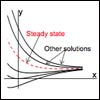# First Order Constant Coefficient Linear ODE's

« Previous | Next »

## Session OverviewIn this session we focus on constant coefficient equations. That is, the equation y’ + ky = f(t), where k is a constant. Since we already know how to solve the general first order linear DE this will be a special case. Studying it will pave the way for studying higher order constant coefficient equations in later sessions.

## Session Activities

Read the course notes:

Watch the lecture video clip:

Read the course notes:

Watch the lecture video clips:

Read the course notes:

Watch the problem solving video:

« Previous | Next »

#### Learning Resource Types

theaters Lecture Videos
theaters Recitation Videos
grading Exams with Solutions
laptop_windows Simulations
notes Lecture Notes
assignment_turned_in Problem Sets with Solutions